• Call Now

1800-102-2727•

# Reflection of light

Let's play an interesting game. First, keep your face in front of a dull wall and observe. Now see your face on your shining floor and note the observation. Now take a bucket of water and see your face in it and observe. At last, see your face in the plane mirror at your home. If you compare the observations of all four cases you will find there is no image of your face when you see it on the wall. There is an image with very poor quality when seen on the floor. When you see the face in the water still you can see the image but not clear. You got a proper image of good quality when seen in the plane mirror. Although all four are plane surfaces, still we are getting different quality images. This phenomenon can be understood by the reflection of light. The walls of the house are rough in nature so produce diffuse reflection and regular reflection is very less when light falls on it. On the other hand, the mirror reflects almost all the light that falls on it. Let's learn more about the reflection of light!

Table of content

• Reflection of light
• Laws of Reflection
• Terminology used in reflection of light
• Object and image
• Practice problems
• FAQs

## Reflection of light

When a light ray encounters a boundary between two different media and bounces back to enter the same medium, this phenomenon is referred to as the reflection of light. The ray which falls on the surface is called the incident light ray. The ray that bounces back is known as the reflected ray. If we draw a perpendicular on the reflecting surface, at the point of incidence it is called the normal. The point where a light ray coming from an item or source appear to meet after being reflected by a surface is called an image. We can see our surroundings because light reflects off a variety of surfaces.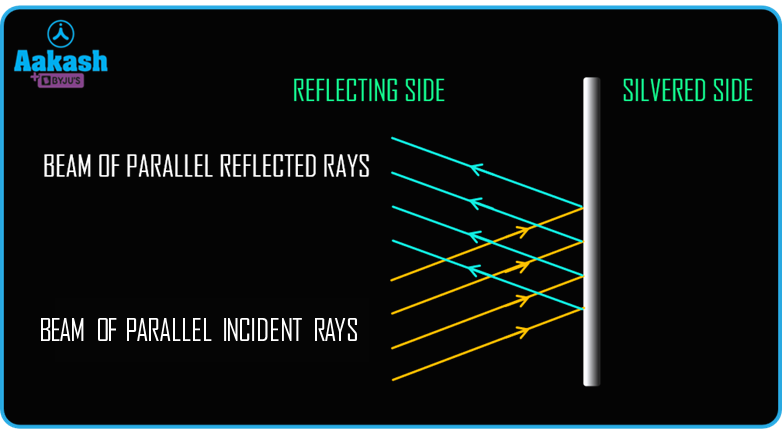The reflecting surface can be plane or spherical. In the above figure, a plane reflecting surface is shown. A parallel beam of light incident on the surface after the reflection is bounced back in the same medium.

## Laws of Reflection

There are two laws that govern the reflection of light (reflected from any surface). These Laws are referred to as laws of reflection. When light from any object reflects from polished surfaces, the reflection is called regular reflection. Regular reflection produces an image. We obtain two different types of images: real and virtual, depending on the mirror (reflecting surface) and object position.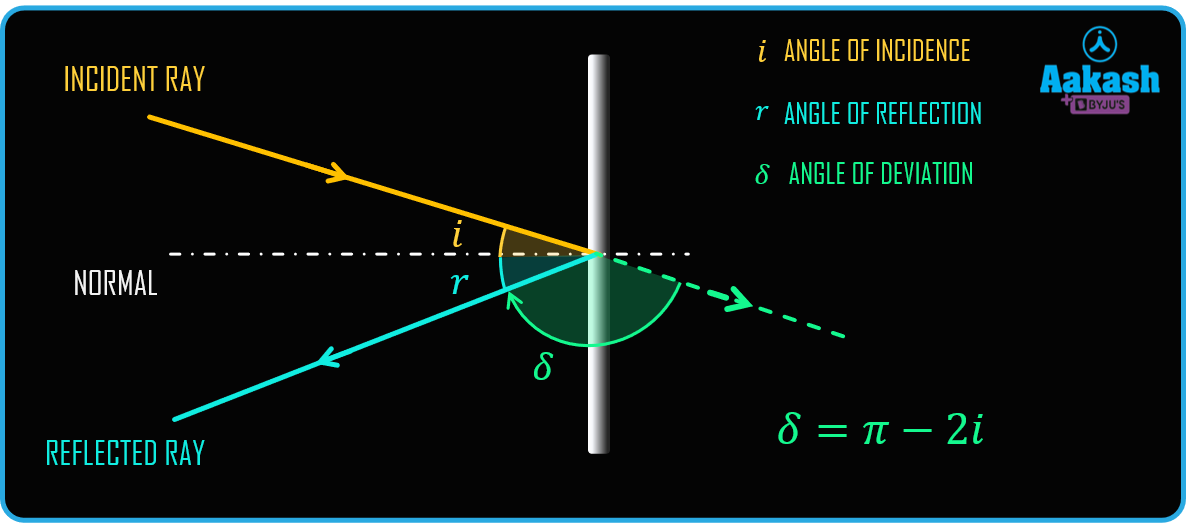At home, we create our virtual image using plane mirrors. The image distance from a plane mirror will be equal to the object distance from the mirror. The light ray is reflected by a planar surface and is subject to the following two laws of reflection.

1. The angle of incidence and reflection is the same. i.e. (i = r).
2. At the point of incidence, the normal to the reflecting surface, the incident ray, and the reflected ray all are coplanar.

## Terminology used in reflection of light

1. Incident ray : A light beam that strikes the mirror after emerging from an object or the source.
2. Reflected rays : The light beam that bounces back after striking a reflective surface.
3. Point of incident : It is the location where the incident ray makes contact with the surface.
4. Normal : It is the line which is perpendicular to the reflecting surface at the point of incident.
5. Angle of incidence : Angle of incidence is the angle formed at the point of incidence between the incident ray and the normal to the surface. It is represented by i.
6. Angle of reflection : Angle of reflection is defined as the angle formed by the reflected ray and the normal to the surface at the point of incidence. It is represented by r.
7. Angle of deviation : Angle of deviation is the angle formed by the directions of the incident and reflected rays. Angle of deviation is given by δ=π-(i+r)=π-2i

## Object and image

Object : An object is everything which is viewed. Also it is the point of intersection of the incident rays. The object can be real and virtual. When the incident rays actually meet (or emerge from) the object is called a real object (figure 1). When the incident rays appear to meet (or emerge from) then it is called a virtual object (figure 2).

Image : The point of intersection of reflected rays is called image. It can also be real and virtual. If the reflected rays appear to meet then it is called a virtual image (figure 1). If the reflected rays actually meet at a point then it is called real image (figure 2).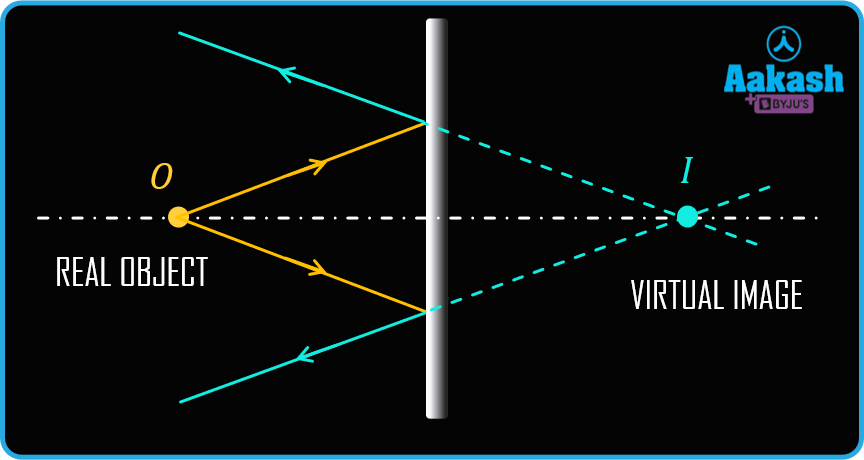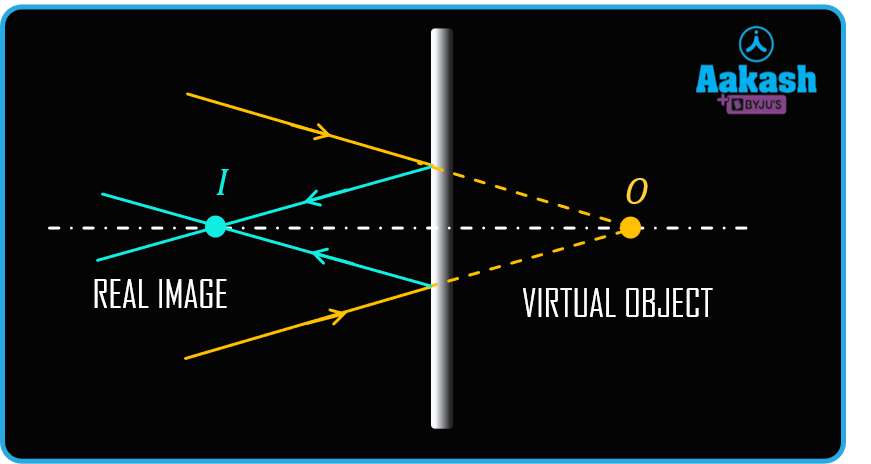### Types of reflection

The reflection of light can be of the following three types :

1. Regular/ Specular Reflection: A smooth surface, such as a mirror, a polished metal, or a calm body of water, will reflect all the light that arrives from a given direction at the same angle. This reflection is known as regular or specular reflection. The image formed is crisp and clear. All the rays of light that are reflected from a surface are parallel if a parallel light source is incident on it. Thus, a regular reflection produces an image. Hence a flat, plane mirror creates an image that is virtual, upright and the same size as the object.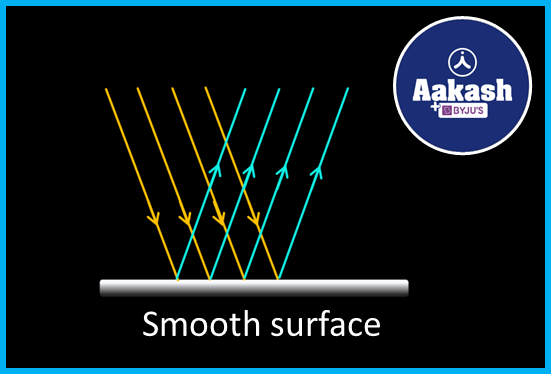1. Diffused Reflection: When light strikes rough surfaces, it reflects in a diffuse manner. When a beam of light strikes such a surface, it is reflected at different angles and in all directions. Depending on how rough their surfaces are, the light that strikes the objects in our room is diffused in all directions. This reflection does not produce any image.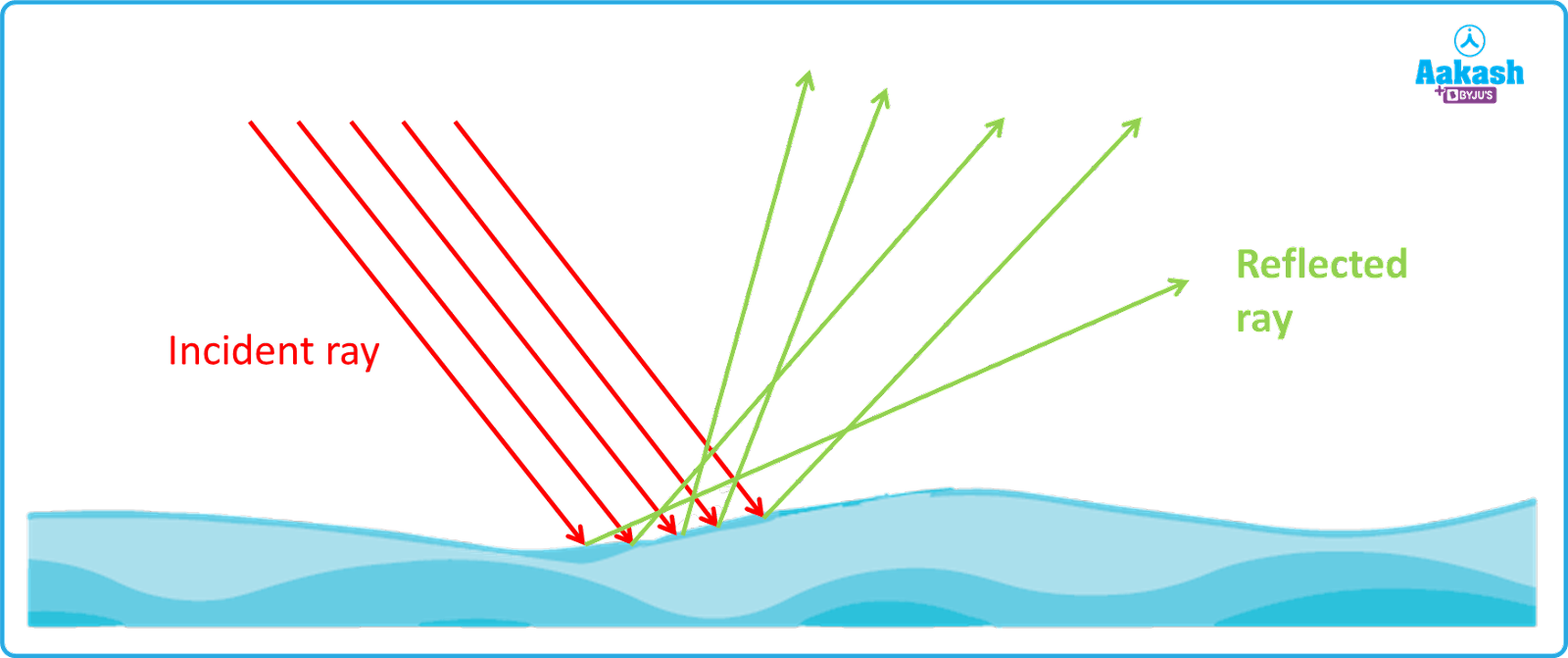1. Multiple reflection : Multiple reflections is similar to regular reflections but it uses more than one mirror. Multiple reflections occur in these situations when two mirrors are positioned at an angle. The angle formed by the two mirrors determines how many images are visible.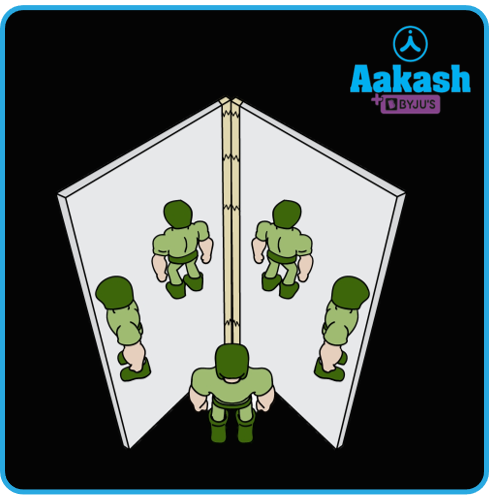Application of reflection of light in our daily life

The most general application of reflection of light is given below :

• Reflection of light on the polished surface.
• Periscopes use reflection of light to let forces see oncoming enemies on the battlefield without endangering their safety.
• Medical diagnostics and optical communications are made possible through reflection of light.
• We can see the moon because the light rays coming from the sun get reflected from the surface of the moon.
• The law of reflection makes it possible to precisely determine distances between objects.
• We can see the colours of diverse objects through reflection of light.

## Practice problems

Q1. A light ray is incident on a plane surface at an angle 37o with the surface, what is the angle of reflection?

A. Given angle with the surface $\theta$s = 37o

The angle of incidence i = 90- $\theta$= 90- 37= 53o

As per the law of reflection, angle of incidence is equal to angle of reflection i = r

So the angle of reflection= 53o.

Q2. The angle of incidence for a plane reflecting surface is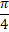. What is the angle of deviation?

A. Given angle of incidence $i=\frac{\pi }{4}$

The deviation's angle is given by,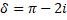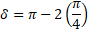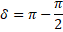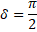The angle of deviation is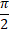or 90o.

Q3. Write the characteristics of the image formed by the plane mirror?

A. The characteristic of the image formed by plane mirrors are as follows :

1. The image and the object from the mirror are separated by the same amount of distance.
2. The image formed is virtual and upright.
3. The image is the exact same size as the object.
4. The image produced is laterally inverted i.e. right is shown as left, and left as right.

Q4. A reflecting surface deviating the light ray at an angle 120o. What is the angle of incidence?

A. Given $\delta ={120}^{o}$
The angle of deviation is given by,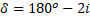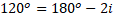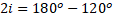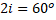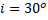Hence the angle of incidence is 30o

## FAQs

Q1. Which of the following cases the laws of reflection hold good for?

1. plane mirror
2. concave mirror
3.convex mirror
4. all reflecting surfaces.

A. The law of reflection is valid for all the reflecting surfaces including plane mirrors, concave mirrors and convex mirrors. Concave and convex mirrors have curved surfaces but at the microscopic level it can be considered to consist of an infinite number of a plane reflecting surfaces.

Q2. How can you see the reflection of light on the moon?

A. The light coming from the sun falls on the moon and after the reflection from the moon it reaches the earth and thus we can see it. The portion on which the light falls appears bright and the rest appears dark.

Q3. Which object reflects more light?

A. Compared to objects with dull or unpolished surfaces, glossy or polished objects reflect more light. The best light reflector is made of silver metal.

Q4. Can water reflect the light?

A. Yes, water can reflect light. Also, it refracts the light. When the light falls on the water surface some of the light gets reflected and some refracted into it.Talk to our expert
Resend OTP Timer =
By submitting up, I agree to receive all the Whatsapp communication on my registered number and Aakash terms and conditions and privacy policy